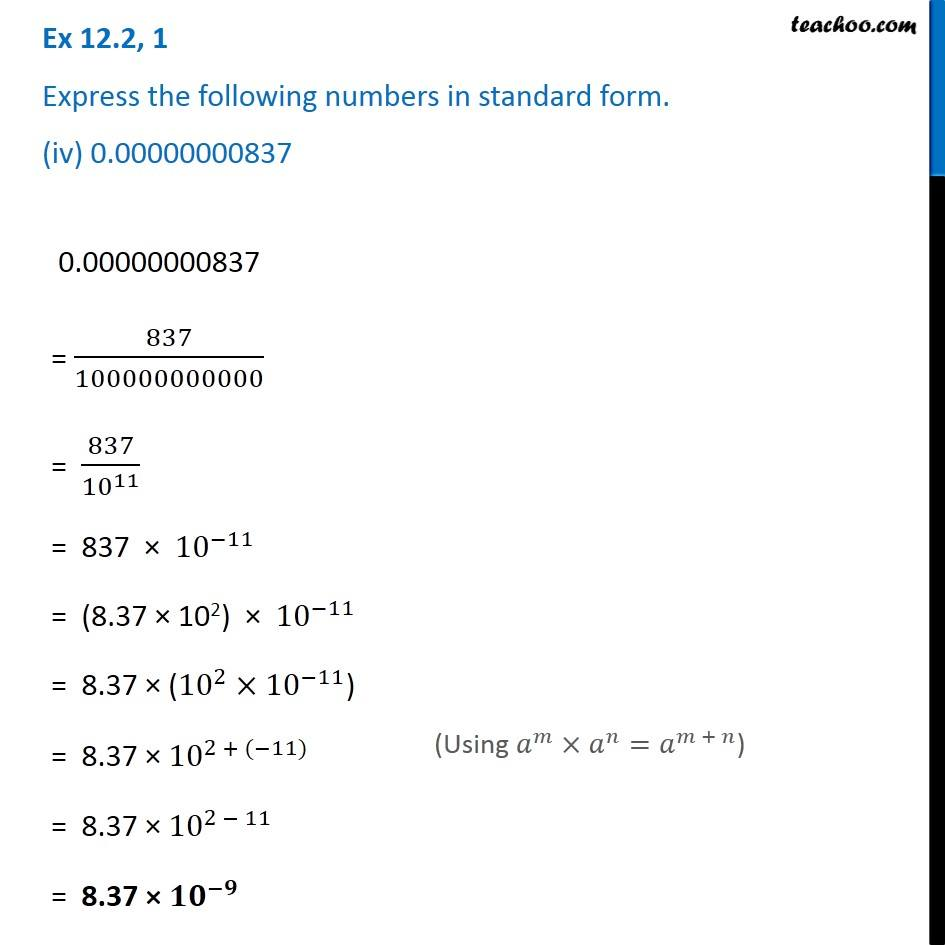Ex 12.2

Chapter 12 Class 8 Exponents and Powers
Serial order wiseIntroducing your new favourite teacher - Teachoo Black, at only ₹83 per month

### Transcript

Ex 12.2, 1 Express the following numbers in standard form. (iv) 0.00000000837 0.00000000837 = 837/100000000000 = 837/〖10〗^11 = 837 × 〖10〗^(−11) = (8.37 × 102) × 〖10〗^(−11) = 8.37 × (〖10〗^2×〖10〗^(−11)) = 8.37 × 〖10〗^(2 + (−11)) = 8.37 × 〖10〗^(2 − 11) = 8.37 × 〖𝟏𝟎〗^(−𝟗) (Using 𝑎^𝑚×𝑎^𝑛=𝑎^(𝑚 + 𝑛))